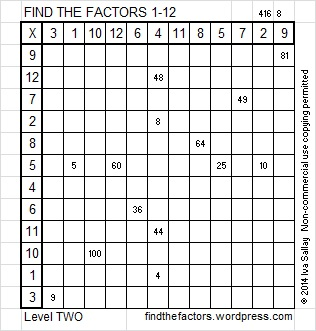97 and Will This Six-legged Puzzle Bug You?

• 97 is a prime number.
• Prime factorization: 97 is prime.
• The exponent of prime number 97 is 1. Adding 1 to that exponent we get (1 + 1) = 2. Therefore 97 has exactly 2 factors.
• Factors of 97: 1, 97
• Factor pairs: 97 = 1 x 97
• 97 has no square factors that allow its square root to be simplified. √97 ≈ 9.8488578How do we know that 97 is a prime number? If 97 were not a prime number, then it would be divisible by at least one prime number less than or equal to √97 ≈ 9.8. Since 97 cannot be divided evenly by 2, 3, 5, or 7, we know that 97 is a prime number.

97 is never a clue in the FIND THE FACTORS puzzles.This week’s puzzles and last week’s solutions: 12 Factors 2014-04-21This site uses Akismet to reduce spam. Learn how your comment data is processed.